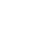# How to find Neutral Current in 3 phase system?

8
13677This is the simple calculator to find neutral current in three-phase system. Often you need to find neutral current to size the neutral conductor. Also, in case of neutral conductor overheating you need to know that how much current is flowing through neutral.

Remember that in balanced three-phase system the current through neutral is zero. However, practically this is not always the case.

### How to use the calculator?

• Enter the current drawn in all three-phases. You can note down the current flowing through each phase from Multi-function meter located on your panel. Another option is, you can individually measure current in each phase with the help of clamp-on meter.

### How to find neutral current?

You can also use below formula to find neutral current,Also See other Useful Calculators

1.Davide Tagliafierro

Hello, the formula at the bottom has some type errors.
It should be
Sqrt( iR^2 + iY^2 + iB^2 – iR*iY – iR*iB – iY*iB )

•Krunal Shah

Thank you my dear friend. I have removed that error.

2.Balamurugan

Sir,
As we have connected a DT in agri feeder (I.e.) some times 3 phase supply and some times 2 phase supply-{RYY}, and hence the neutral current is so high. Will the DT may failed if continued in service? Pl tell me.

•Krunal Shah

Yes, it may harm your DT. Single phase loads running on DT should be distributed evenly in each phase.

3.Mark Menegon

•Krunal Shah (Mod)

If you want to calculate power factor, check out this link
Power Factor Calculator
If you want to improve power factor, check out this calculator,
Power Factor Correction Calculator

4.Md afroz alam

I wanted to know that the ampere is in neutral

•Krunal Shah